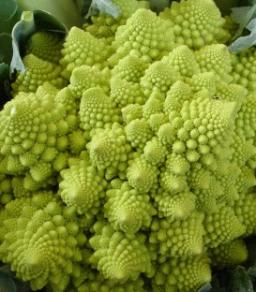# Teachers 4379

The hostel has 90 people; There are three times more boys than girls, and there are 70 fewer teachers than boys and girls together. How many teachers are there?

u =  10

### Step-by-step explanation:

u+a+b=90
a = 3b
u = a+b-70

u+a+b=90
a = 3·b
u = a+b-70

a+b+u = 90
a-3b = 0
a+b-u = 70

Row 2 - Row 1 → Row 2
a+b+u = 90
-4b-u = -90
a+b-u = 70

Row 3 - Row 1 → Row 3
a+b+u = 90
-4b-u = -90
-2u = -20

u = -20/-2 = 10
b = -90+u/-4 = -90+10/-4 = 20
a = 90-b-u = 90-20-10 = 60

a = 60
b = 20
u = 10

Our linear equations calculator calculates it.Did you find an error or inaccuracy? Feel free to write us. Thank you!

Tips for related online calculators
Do you have a system of equations and looking for calculator system of linear equations?